# Is the Rossi energy amplifier the first pico-chemical reactor?

by
Jacques Dufour
CNAM Laboratoire des sciences nucléaires, 2 rue Conté 75003 Paris France

Abstract:
The nuclear signatures that can be expected when contacting hydrogen with nickel, were derived from thermal results recently obtained (Rossi energy amplifier), using the type of reaction paths proposed as the explanation of the energy produced. The consequences of proton or neutron capture have been studied. It was shown that these consequences are not in line with the experimental observations. A novel tentative explanation is thus described. Should this explanation be true, it is proposed to call pico-chemistry the novel field thus opened.
Introduction:
In a recent paper , it was shown that, if the reaction path occurring in a Rossi energy amplifier , was mainly proton capture, the lead thickness required to completely suppress the gamma flux produced, would be in the order of tens of cm. The lead screen used (2 cm) should thus have resulted in a lethal gamma dose emitted in the surroundings. Another explanation, different from proton or neutron capture is thus to be found. In , the concept of pico-chemistry was presented, that could explain the generation of photons in the range of tens of keV, thus compatible with the lead screening used in the energy amplifier.
In chemistry, compounds are formed by the binding of the components through their outer electronic shells. Ionic, metallic and covalent hydrides of metals are known. Thus, Nickel hydride NiH can be viewed as an hydrogen and a nickel atoms maintained at a few angstrom distance, through a metallic bound.
In contrast, in a pico-nickel hydride, a (shrunken) hydrogen atom would be inside the electronic cortege of the Nickel and bound to the nickel at close proximity of its nucleus. In  a tentative explanation was given, of the possibility of such an exotic hydride. Another approach is presented in this paper.

Possible existence of a small hydrogen-like dipole and reaction with a nickel nucleus:
Various concepts of a shrunken hydrogen atom have been presented. In , the possibility of having bound states of a proton and an electron with lower radius and higher ionization energy than the usual Bohr values is claimed. These bound states were called hydrinos and attributed to the possibility of having fractional values for the main quantum number of the hydrogen atom. In  a metastable state is justified by the electron spin/proton nuclear spin interaction being first order in the environment of a lattice (it is third order in vacuum). This state was called hydrex and proposed as an explanation for fission-like reaction occurring in metallic lattices. Finally, the interaction of a proton and an electron could result in a virtual neutron , that could be captured by and react with the Ni nucleus.
In this paper, the evolution of a virtual neutron like association between a proton and an electron, in contact with an atom is examined.
At the surface of various materials (metals, metal oxides, metal hydrides…), electrons are more or less free to leave the solid (work function). In an hydrogen environment, it is conceivable that from time to time a virtual neutron can be formed between such an electron and a proton , with a deficit of energy of 0.781 MeV: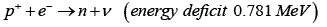The life time of this virtual neutron is limited by the Heisenberg uncertainty relation ∆t∆E>h, which sets the maximum distance d it can travel: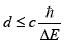The maximum of d is thus some 250 fm and the virtual neutron, formed at the periphery of an atom has hardly any chance to reach the close vicinity of the nucleus of this atom. It can nevertheless sufficiently penetrate the outer electronic cortege of the atom so as to feel the (screened) positive potential of the nucleus of the atom, when reverting to a proton and an electron. An electrical dipole is thus formed, which is attracted by the nucleus of the atom. One can wonder if the resulting effect of the action of the positive charge of the nucleus will ultimately end up in the destruction of the dipole, the proton being rejected to infinite and the electron bound to the nucleus. This would certainly be the case if the nucleus where not surrounded by its electronic cortege (Z time ionized nucleus). In the case of an atom with its electrons, an equilibrium position of the dipole could be reached, at close vicinity of the nucleus. To demonstrate the possibility of such a bound state, the complete Hamiltonian of the system would have to be solved, which is not possible. A semi-empirical approach has thus been developed, to reach the orders of magnitude of the characteristics of such a dipole and its interactions with an atom A. This could be used as a guide when looking at the experimental results expected in case of an excess energy measured in the system hydrogen/nickel (energy of radiations emitted, characteristics of the by-products).
In order to distinguish this concept of shrunken hydrogen atom from others, it is proposed to call it Hypole (Deupole and Tripole being the 2 other isotopes).

Semi empiric description of the Hypole: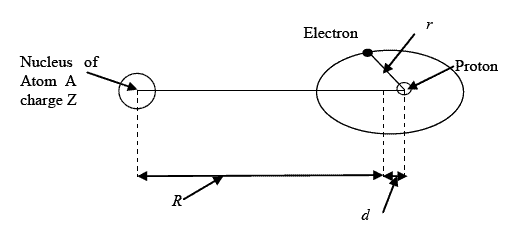Figure 1 gives a description of the Hypole, which is proposed to be written H¯Ni when the host atom A is Nickel and its (possible) bound state with the Ni atom, a Nickel pico-hydride NiH¯Ni.
d is the distance between the centers of gravity of positive and negative charges in the hypole.
r is the distance between the proton and the electron.
R is the distance between the center of the nucleus of the atom A and the center of gravity of the hypole.
Z is the charge number of the atom A
The potential that the dipole proton/electron feels from A is at first order (when d/R is small):During its attraction by A, the spatial extension of the dipole is limited by the repulsion of the inner layers of the electrons of A, resulting in a shrinking of this hydrogen-like object and in a limitation of its polarization. In order to get first guesstimated values of the size and energy of the hypole and of the bound state it might form with A, following assumptions are made:
1. The action of the electronic cortege of A (especially the s electrons of A) on the dipole and the presence at short distance of the Z protons of A are equivalent to the attraction of the electron by the proton in the hypole being multiplied by a factor K>1. Hence, the (pseudo) coulomb interaction in the dipole is:2. d is small and proportional to R. Hence, d=kR, with k small.
3. The electron of the hypole H¯A cannot be found in the nucleus of the atom A (competition with the s electrons of A). Hence, r≤R
With these assumptions, the Bohr radius of H¯A would be: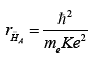and its energy of formation: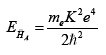In a similar way, the Bohr radius of AH¯A would be: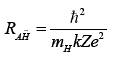and its energy of formation: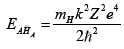with mH being the mass of the hydrogen atom.
Under assumption 3, the smallest possible bound object AH¯A is obtained for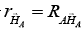In that case meK=mHKZ. Expressing the energies as a function of the unknown k, one gets:and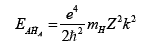finally yielding the following value for the total energy given by the hypole formation followed by its binding with A:The bulk of the energy is coming from the formation of the Hypole. EH¯A likely to be of the order of magnitude of the energies that can be found close to the A nucleus, that is the s electrons energy E^sA.
A guesstimated value of k is thus: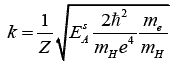In the case of Nickel and taking for E^sNi the average value 10.5 keV, the following guesstimated description of H¯Ni and NiH¯ Ni is obtained (Table 1):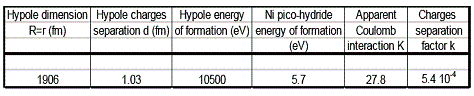Properties of the Hypole:
The hypole is a picometer size hydrogen-like object. It can only exist when embedded in the electronic cortege of an atom A, where its equilibrium position is very close to the nucleus of A. Its size and energy of formation depends upon A. In the case of Nickel, the size is some 2 picometer and the energy of formation round 10 keV. Hence the names and notations proposed.

The best way for characterizing an hypole, is to measure the mass of the corresponding A/pico-hydride. In the case of nickel, following masses are expected, that take into account the energy of formation (Table 2):The mass differences given by Table 2 could be easily detected using a high resolution TOF Mass Spectrometer on an acidic solution of the nickel pico-hydride (probably possible see below, chemical properties). SIMS TOF Mass Spectrometry is not adapted, since the primary ions energies are of the order of the energy of formation of the hypole. An ICP TOF Mass Spectrometer would be adapted.

As regards the chemical properties of NiH¯Ni, they should be close to the Nickel ones. The outer electronic layers of NiH¯Ni indeed see the positive charge of the nickel atom, the effect of the hypole H¯Ni being second order in that respect. A shift of the characteristic rays given by nickel in ICP-AOS could be observed.
Finally the radiations emitted during the hypole formation, would be photons in the 10 keV range, thus completely suppressed by the 2 cm layer of lead in the energy amplifier. Faint signals of higher energy photons (annihilation radiation) could anyhow be detected. They might be the signature of an inherent instability of the hypole and of the corresponding pico-hydride, which is discussed now.

Stability of the (nickel) hypole:
The nickel hypole is a small object of picometer dimension and at picometer distance from the nickel nucleus. Its virtual neutron state may have a non zero probability to penetrate the nickel nucleus and react with it according to the neutron capture route developed in  and . Most of the gamma photons resulting from the stabilization of the primary excited nickel nuclei are of energy higher than 1 MeV . They mainly interact with the lead shield by producing electron/positron pairs, ultimately yielding the annihilation radiation. From the experimental observations, the rate of virtual neutron capture should be very low (some 10^-20 s^-1, in the experiment 2009(3-5/4-26) presented in ).

Conclusions:
In this paper, a rough description is given, of a novel chemical interaction. Orders of magnitudes of the main characteristics of this still hypothetical interaction are given.

It is hoped that this approach will be of help when trying to understand the thermal results obtained with the energy amplifier.
Should the experimental results and their interpretation be true, pico-chemistry would become a reality.

References:
 J. Dufour “Nuclear signatures to be expected from Rossi energy amplifier” Journal of nuclear physics May 6th 2010
 S. Focardi and A. Rossi “A new energy source from nuclear fusion” Journal of nuclear physics
 J. Dufour “Very sizeable increase of gravity at pico-meter distance: a novel working hypothesis to explain anomalous heat effects and apparent transmutations in certain metal hydrogen systems” J. of condensed matter nuclear science 1 (2007) p 47-61.
 R.L. Mills and W.R. Good “A unified theory derived from first principles” Black light power, Inc. (1992)
 J. Dufour, D. Murat, X. Dufour and J. Foos “Experimental observation of nuclear reactions in palladium and uranium: possible explanation by the hydrex mode” Fusion Science and Technology Vol.40-July 2001- p.91-106
 L. Daddi “Virtual neutrons in orbital capture” Journal of nuclear physics March 18, 2010

### 102 comments to Is the Rossi energy amplifier the first pico-chemical reactor?

• Andrea Rossi

Joseph:
Yes,
Warm Regards,
A.R.

• Joseph

do you think the certification will go through ?